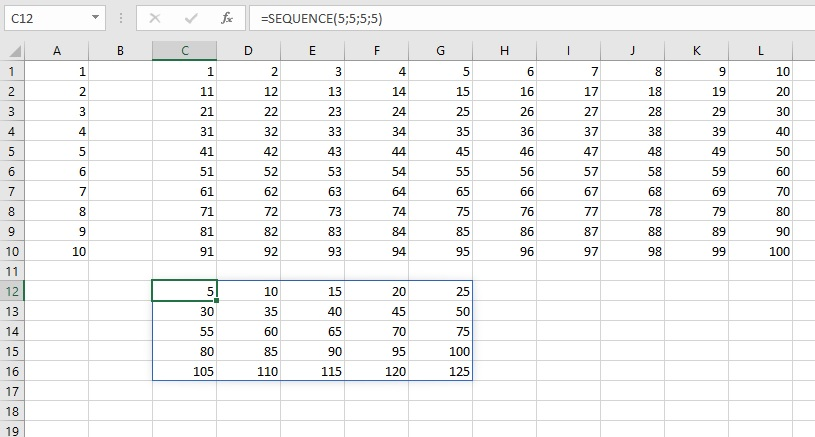# SEQUENCE function

The SEQUENCE is another function that manipulates dynamic arrays. It allows generation of an array of numbers within a vector or matrix, depending on the given parameters, and it is possible to set the initial value as well as the step value by which the series is being incremented. So, it’s something like when you use the Fill Series option, except that you can now do it with the function.

The syntax of the SEQUENCE function is:

SEQUENCE (<rows>,[<columns>],[<start>],[<magnification>])

The first argument is the number of rows and the second is the number of columns within which a series of numbers is generated. The number of rows is mandatory and the number of columns is an optional parameter. The start is also optional, as well as the step value.

If we write the formula:

=SEQUENCE(10)

the result will be a dynamically generated series in the area A1: A10 with values ​​from 1 to 10. If we write the formula:

=SEQUENCE(10,10)

a 10×10 size matrix with numbers from 1 to 100 will be constructed.

By specifying the third parameter, we can determine from which value ​​the queue begins to grow, and by specifying the step value we determine the value of increment in each successive step. By creating a formula:

=SEQUENCE(5,5,5,5)

we construct a 5×5 matrix that starts from number 5 and ends with number 125. For now, this feature is only supported within Office 365 plans.